# 20191113 2019-2020-2 《Python程序设计》实验二报告

## 1.实验内容

• 设计并完成一个完整的应用程序——计算器。包含：
• 简单四则运算
• 取模运算
• 求简单三角函数
• 求一个数的n次方
• 求一个数的阶乘
• 解一元二次方程（复数域上）
• 统计运算（包括求和、平均值、极差、方差、标准差）
• 练习基本语法、判定语句、循环语句、逻辑运算等知识

## 2. 实验过程及结果

### 明确目的

• 简单四则运算

混合四则运算难度系数过大，目前对知识掌握的熟练程度并不能支持我进行编程。

• 取模运算

• 求简单三角函数

• 求一个数的n次方

• 求一个数的阶乘

• 解一元二次方程（复数域上）

• 统计运算（包括求和、平均值、极差、方差、标准差）

### 设计思路

• 简单三角函数运算

日常输入常以角度为主，Python算法要求弧度制，需先将输入角度化为弧度制，后进行计算。

• 解一元二次方程

在复数域上解一元二次方程包含了实数域上解一元二次方程，但因表示法的不同，应把二者区分开来。

• 统计运算

统计运算中求和作为求平均值的基础，求方差作为求标准差的基础。可以适当进行排序，以求简化计算。

### 编码、调试、运行过程

#### 简单四则运算

• 最初版本，将每一个运算写成函数，再在计算器框架内进行调用，但这样造成代码冗余，没有必要。
def add(a,b):    # 加法运算
return a+b
def sub(a,b):    # 减法运算
return  a-b
def mul(a,b):    # 乘法运算
return a*b
def div(a,b):    # 除法运算，使用前需判断除数是否为0
return a/b

• 加强版本，改进了初级版的缺点，但是还是没法给用户较好的体验，如果输错功能数字则需从初始界面开始。
def ari():
print("1.二元加法运算\n2.二元减法运算\n3.二元乘法运算\n4.二元除法运算\n")
temp = int(input("请依照所要使用的功能输入相应的数字序号："))
if temp == 1 or temp == 2 or temp == 3:
x = float(input("请输入第一个操作数："))
y = float(input("请输入第二个操作数："))
if temp == 1:
print(x + y)
elif temp == 2:
print(x - y)
else:
print(x * y)
elif temp == 4:
x = float(input("请输入被除数："))
y = float(input("请输入除数："))
while y == 0:
print("输入错误！除数不能为0！")
y = float(input("请重新输入除数："))
print(x / y)

• 最终版，添加了while循环，解决如果输入的相应功能数字有误的问题。
def ari():
'''四则运算'''
print("1.二元加法运算\n2.二元减法运算\n3.二元乘法运算\n4.二元除法运算\n")
temp = 233
while temp != 1 and temp != 2 and temp != 3 and temp != 4:
temp = int(input("请依照所要使用的功能输入相应的数字序号："))
if temp == 1 or temp == 2 or temp == 3:
x = float(input("请输入第一个操作数："))
y = float(input("请输入第二个操作数："))
if temp == 1:
print(x + y)
elif temp == 2:
print(x - y)
else:
print(x * y)
elif temp == 4:
x = float(input("请输入被除数："))
y = float(input("请输入除数："))
while y == 0:
print("输入错误！除数不能为0！")
y = float(input("请重新输入除数："))
print(x / y)
else:
print("相应功能数字序号输入有误！")
print("1.二元加法运算\n2.二元减法运算\n3.二元乘法运算\n4.二元除法运算\n")

• 运行结果展示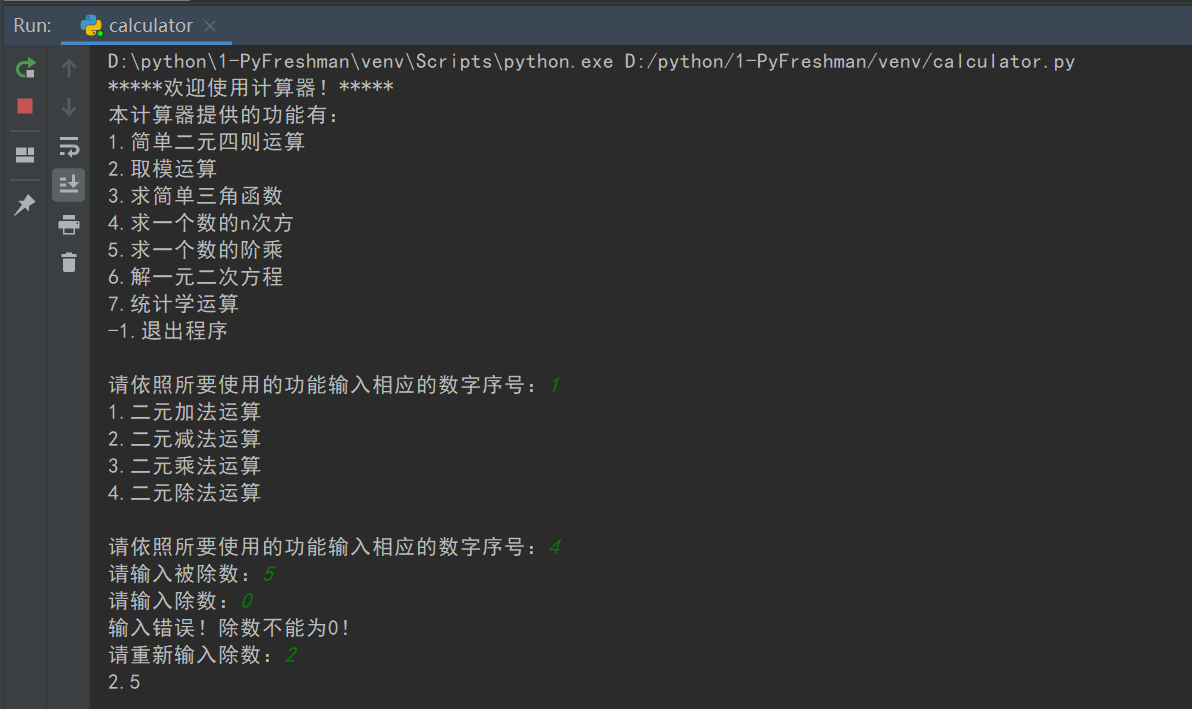#### 取模运算

• 汲取了四则运算的教训，加上运算简单，编起来很是容易。
def mol():
'''取模运算'''
x = int(input("请输入整数被除数："))
y = int(input("请输入整数除数："))
while y == 0:
print("输入错误！除数不能为0！")
y = int(input("请重新输入整数除数："))
print(x % y)

• 运行结果展示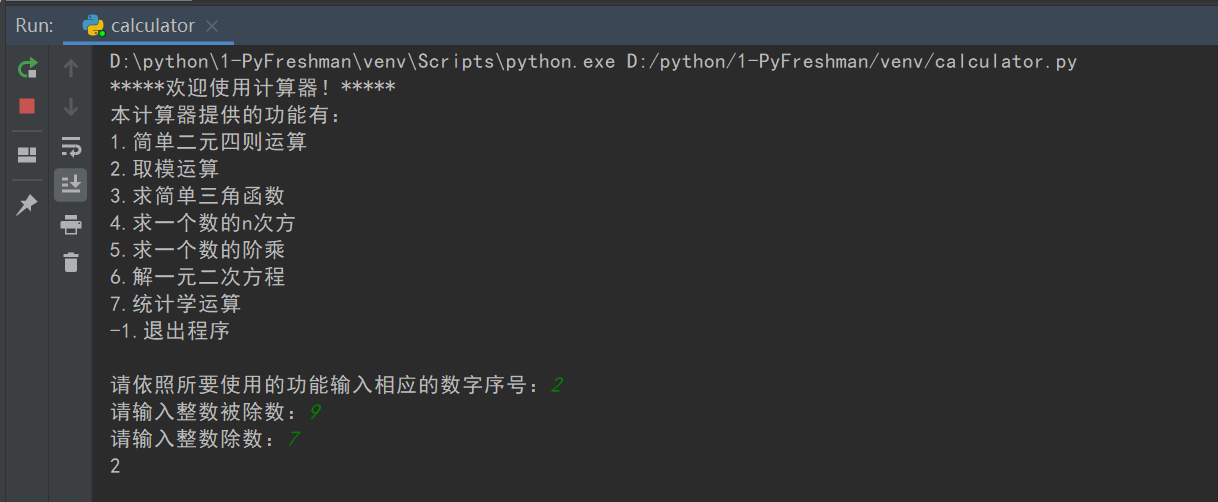#### 求简单的三角函数

• 将六种运算分为两类，先以sin和asin为例，打出简单的代码，进行运行调试。
import math
x = float(input("请以角度制输入角度："))
x = x/180 * math.pi
print(math.sin(x))
x = float(input("请输入相应数值:"))
print('%.2f' % math.degrees(math.asin(x)))

• 以sin和asin的相关代码为模板，进行组装，最后类似简单四则运算进行填充。
def act():
'''求简单三角函数'''
print("\n1.求正弦\n2.求余弦\n3.求正切\n4.求反正弦\n5.求反余弦\n6.求反正切")
temp = 233
while temp != '1' and temp != '2' and temp != '3' and temp != '4' and temp != '5' and temp != '6':
temp = input("请依照所要使用的功能输入相应的数字序号：")
if temp == '1' or temp == '2' or temp == '3':
x = float(input("请以角度制输入角度："))
x = x / 180 * math.pi
if temp == '1':
print('%.2f' %math.sin(x))
elif temp == '2':
print('%.2f' %math.cos(x))
elif temp == '3':
print('%.2f' %math.tan(x))
elif temp == '4' or temp == '5' or temp == '6':
x = float(input("请输入相应数值，答案将以角度制呈现:"))
if temp == '4':
if -1 <= x and x <= 1:
print('%.2f' % math.degrees(math.asin(x)))
else:
print("无解！")
elif temp == '5':
x = float(input("请输入相应数值（-1≤x≤1）:"))
if -1 <= x and x <= 1:
print('%.2f' % math.degrees(math.acos(x)))
else:
print("无解！")
elif temp == '6':
x = float(input("请输入相应数值:"))
print('%.2f' % math.degrees(math.atan(x)))
else:
print("相应功能数字序号输入有误！")

• 运行结果展示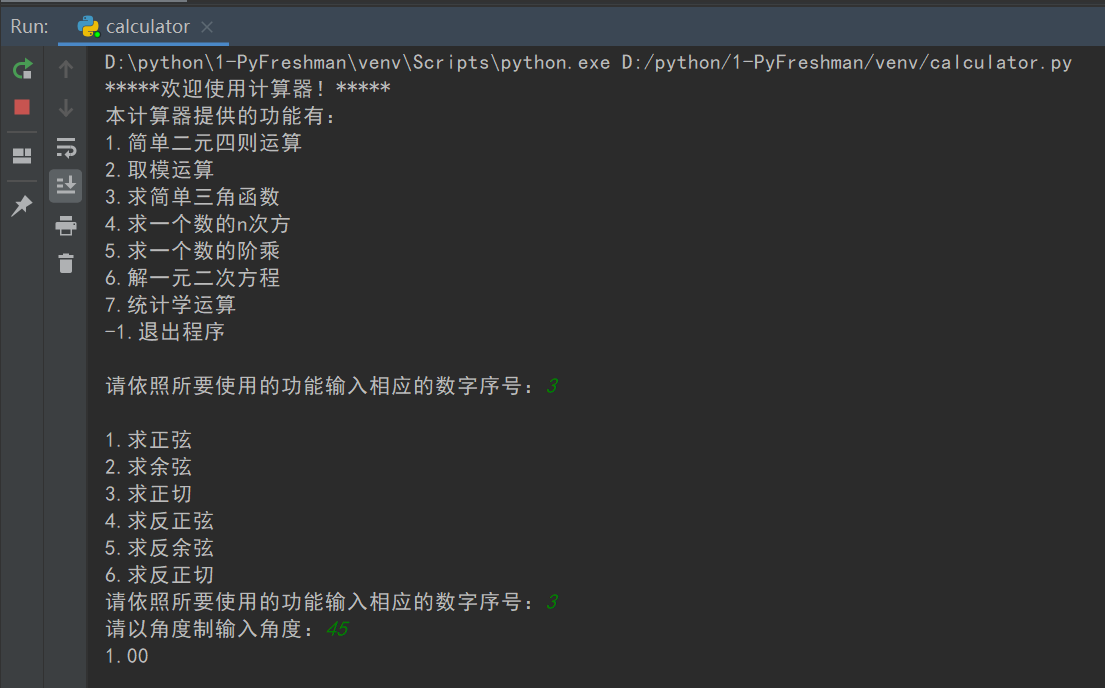#### 求一个数的n次方

• 和取模运算一样代码简单，编码过程中并没有遇到障碍。
def nqo():
'''求一个数的n次方'''
a = float(input("请输入底数："))
while a == 0:
print("输入错误！底数不能为0！")
a = int(input("请重新输入底数："))
n = float(input("请输入指数："))
print(math.pow(a,n))

• 运行结果展示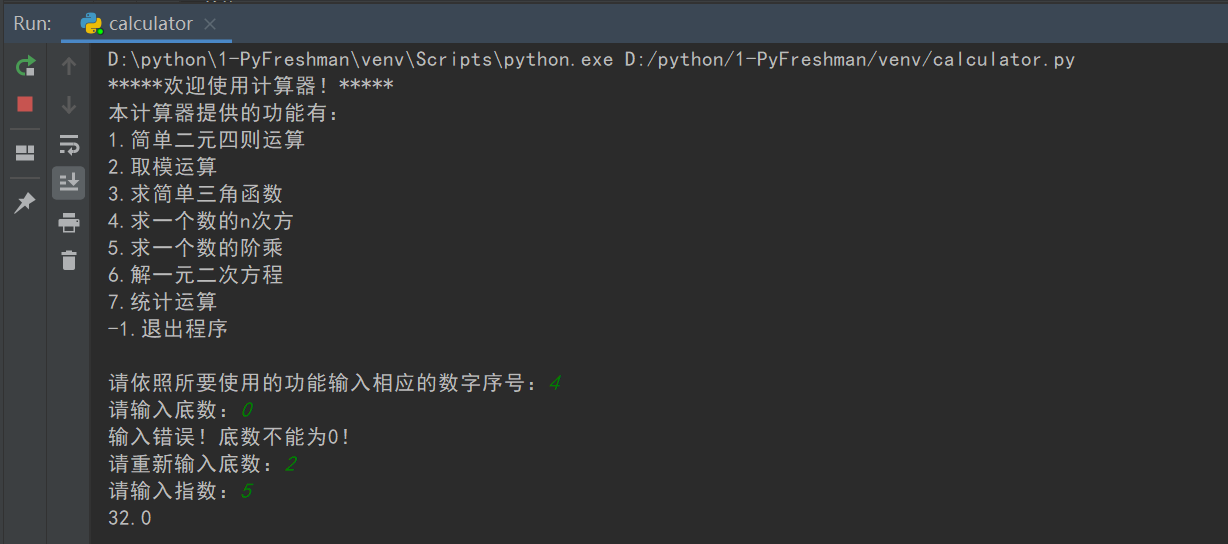#### 求一个数的阶乘

• 一开始不知道有相关的阶乘函数，对其进行了如下编码。
def fac(n):     # 阶乘运算
t = 1
for i in range(1,n+1):
t = t * i
return t

• 发现math库里头的函数又多又实用，对阶乘运算进行重新编码。
def fac():
'''求一个数的阶乘 '''
n = int(input("请输入一个整数："))
print(math.factorial(n))

• 运行结果展示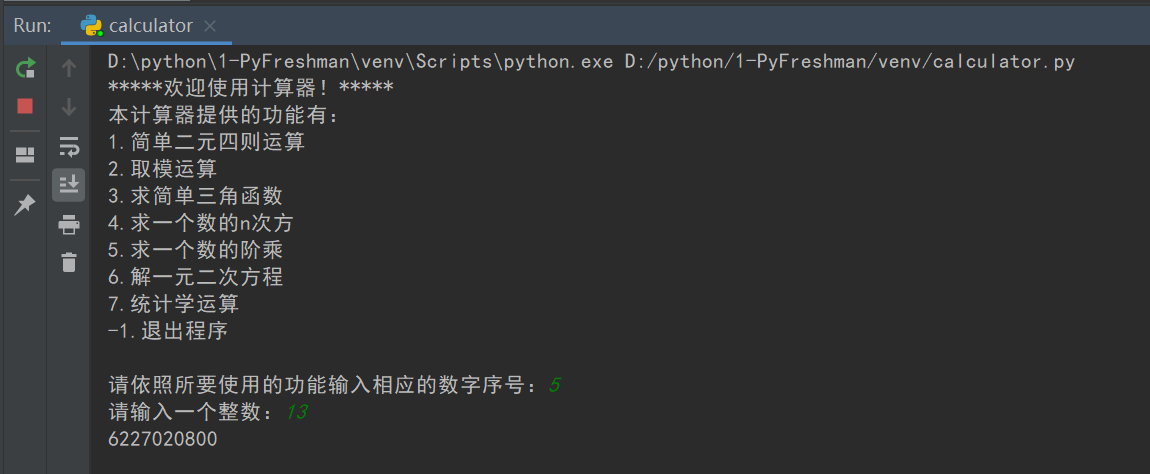#### 解一元二次方程

• 解一元二次方程由于不同情况输出不同的值，在这里用了返回值语句，本打算在计算框架中使用输出语句，但意外的发现，返回值可以直接输出。因此对在返回值行对返回结果进行了格式设置。
def qua():
'''解一元二次方程'''
print('*' * 7 + "解一元二次方程 ax^2 + bx + c = 0" + '*' * 7)
a = float(input("请输入a："))
b = float(input("请输入b："))
c = float(input("请输入c："))
d = b*b - 4*a*c
if d>=0:
x1 = (-b+math.sqrt(d)) / (2*a)
x2 = (-b-math.sqrt(d)) / (2*a)
if d==0:
return "有唯一的解：X = "+str(x1)
else:
return "X1 = "+str(x1)+'\t'+"X2 = "+str(x2)
else:
x1 = str(-b/(2*a)) + '+' + str(math.sqrt(-d)/(2*a)) + 'i'
x2 = str(-b/(2*a)) + '-' + str(math.sqrt(-d)/(2*a)) + 'i'
return "X1 = "+x1+'\t'+"X2 = "+x2

• 运行结果展示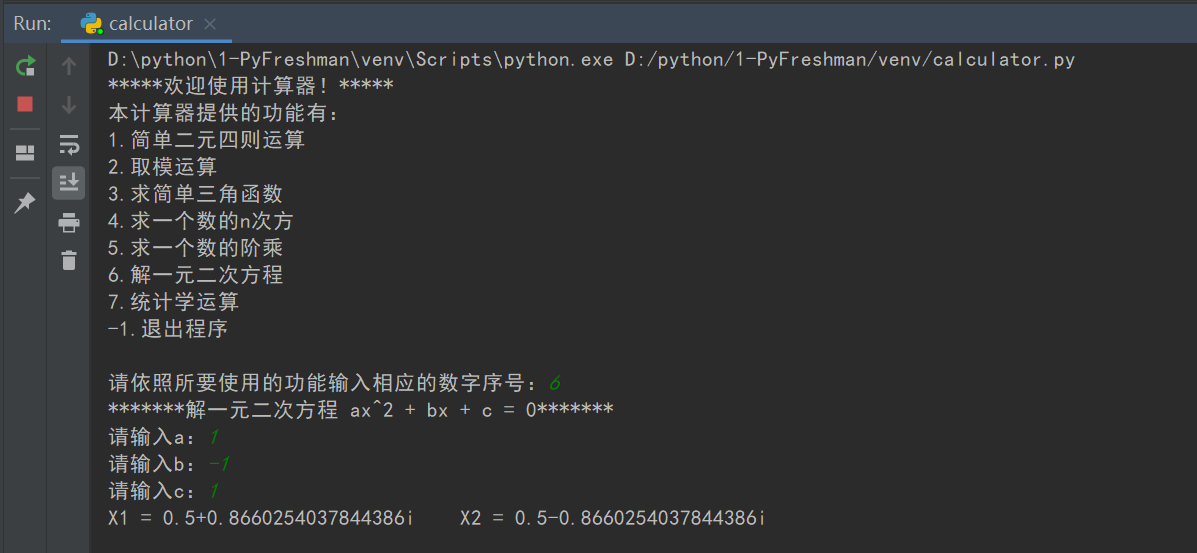#### 统计运算

• 为了进行数据的存储使用了列表，为了对数据进行计算，使用了for 循环。
x = int(input("输入数据个数："))
y = []
print("请输入数据：")
for i in range(x):
temp = [float(input())]
y += temp

• 按照先前所分析的思路将代码编码出，并结合需要编码相关提示语句与循环结构。
def sta():
'''统计运算'''
x = int(input("输入数据个数："))
y = []
print("请输入数据：")
for i in range(x):
temp = [float(input())]
y += temp
print("\n1.求和\n2.求平均值\n3.求极差\n4.求方差\n5.求标准差")
temp = 233
while temp != '1' and temp != '2' and temp != '3' and temp != '4' and temp != '5':
temp = input("请依照所要使用的功能输入相应的数字序号：")
s = 0
for i in range(x):
s = s + y[i]
var = 0
for i in range(x):
var = var + (s / x - y[i]) ** 2
var = var / x
if temp == '1':
print(s)
elif temp == '2':
print(s / x)
elif temp == '3':
print(max(y) - min(y))
elif temp == '4':
print(var)
elif temp == '5':
print(math.sqrt(var))
else:
print("相应功能数字序号输入有误！")


• 运行结果展示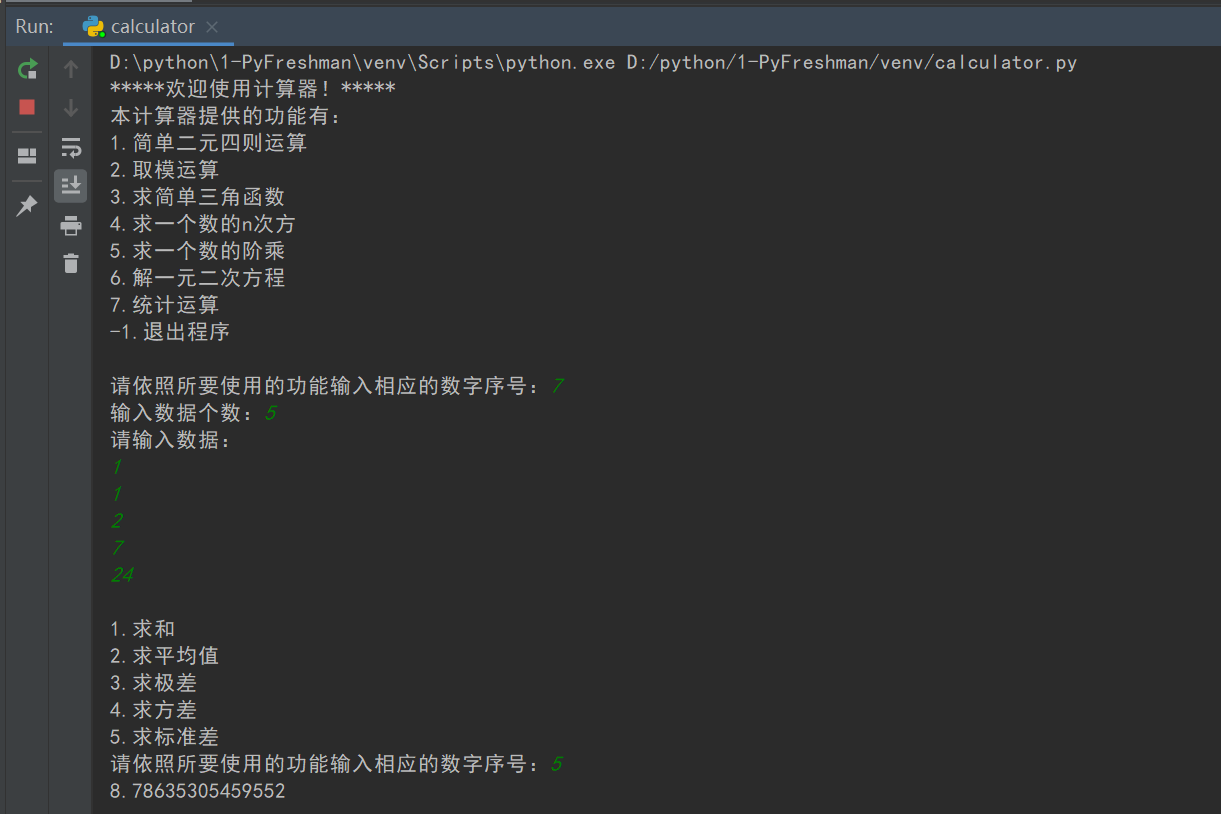#### 计算器框架编码

• 对以上函数进行组装，并加入相关提示语句。为循环，加入while语句，并以“-1”作为程序结束标志。
#计算机功能框架
print('*'*5+"欢迎使用计算器！"+'*'*5)
print("本计算器提供的功能有：")
print("1.简单二元四则运算")
print("2.取模运算")
print("3.求简单三角函数")
print("4.求一个数的n次方")
print("5.求一个数的阶乘")
print("6.解一元二次方程")
print("7.统计运算")
print("-1.退出程序")
t = 233

while t!='-1':
t = input("\n请依照所要使用的功能输入相应的数字序号：")
if t=='1':
ari()
elif t=='2':
mol()
elif t=='3':
act()
elif t=='4':
nqo()
elif t=='5':
fac()
elif t=='6':
print(qua())
elif t=='7':
sta()
elif t=='-1':
exit(0)
else:
print("相应功能数字序号输入有误！")

print("\n是否再次计算？")
print("本计算器提供的功能有：")
print("1.二元四则运算")
print("2.取模运算")
print("3.求简单三角函数")
print("4.求一个数的n次方")
print("5.求一个数的阶乘")
print("6.解一元二次方程")
print("7.统计运算")
print("-1.退出程序")

• 运行结果展示
• 循环
• 终止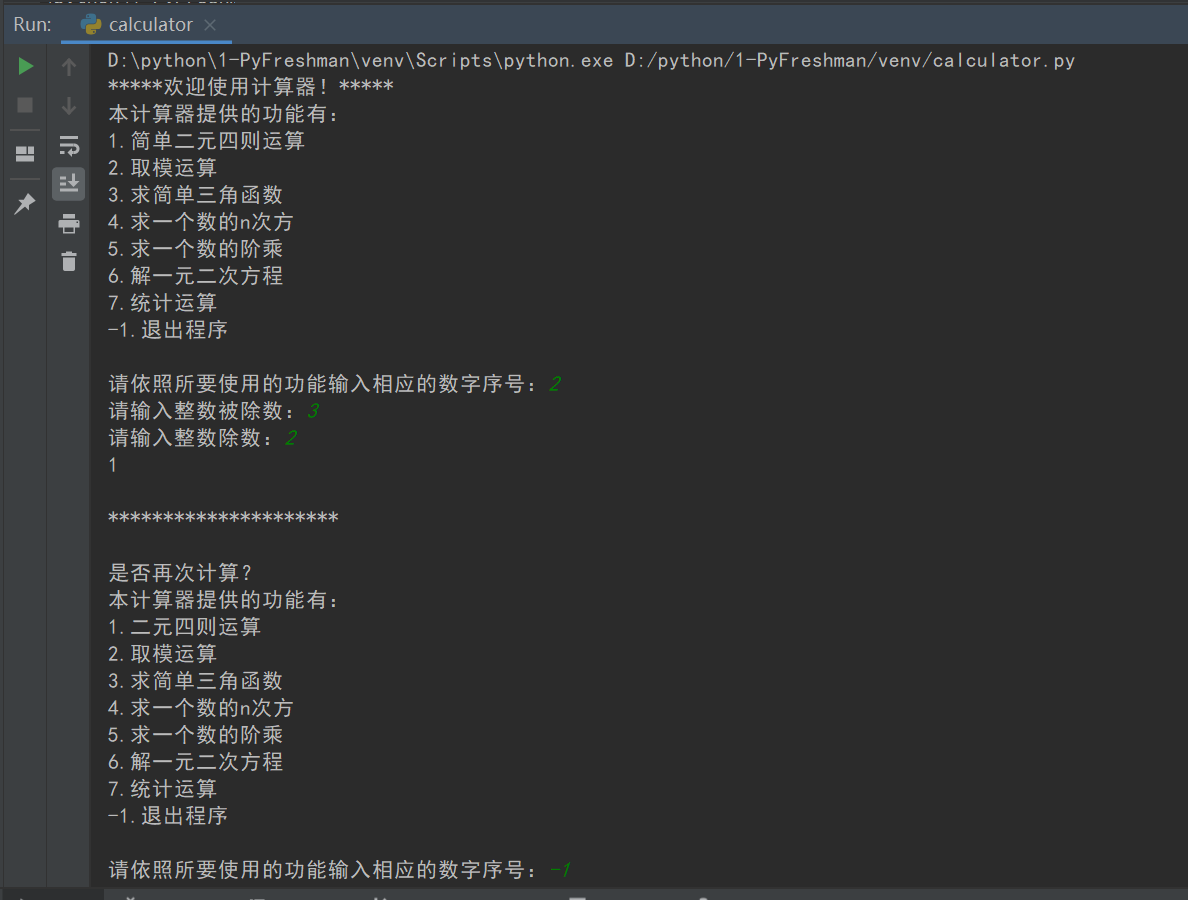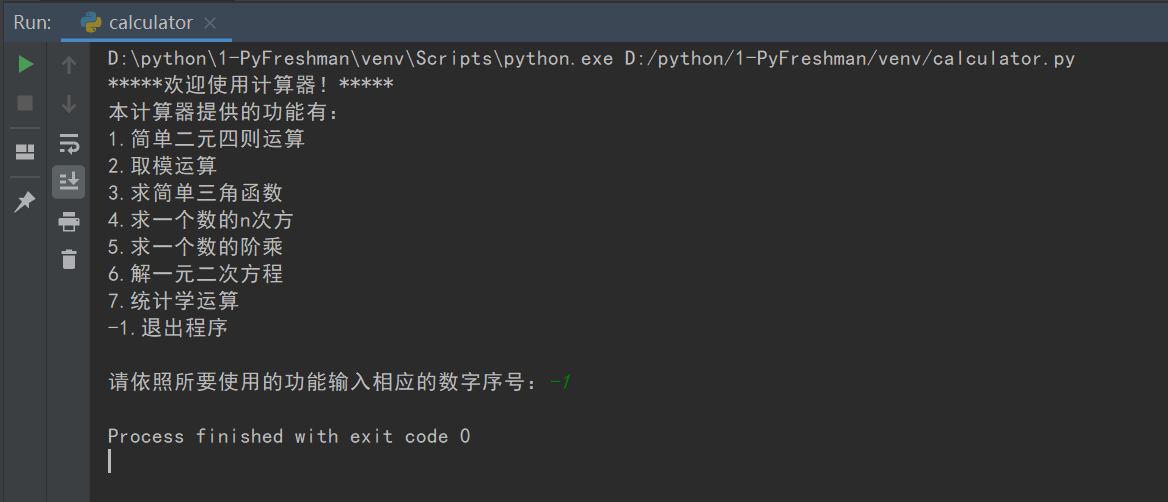## 3. 实验过程中遇到的问题和解决过程

• 问题1：对math.degrees的用法并不熟悉，导致使用错误。

问题1解决方案：查阅相关资料，得知这个函数的用法：从弧度制转化为角度制。若要使角度制变为弧度制应该 x = x/180 * math.pi

• 问题2：在进行求简单三角函数代码编写时，展现出的答案格式不够美观；同时反三角函数类有些答案不对。

问题2解决方案：对答案进行格式限制，同时在输入提示语句中加入输入数值大小限制，同时若输入的数值不在提供范围内，输出“无解!”。

• 问题3：计算器循环结构若想终止，总会将“是否再次运行……”之类不想让其输出的信息输出。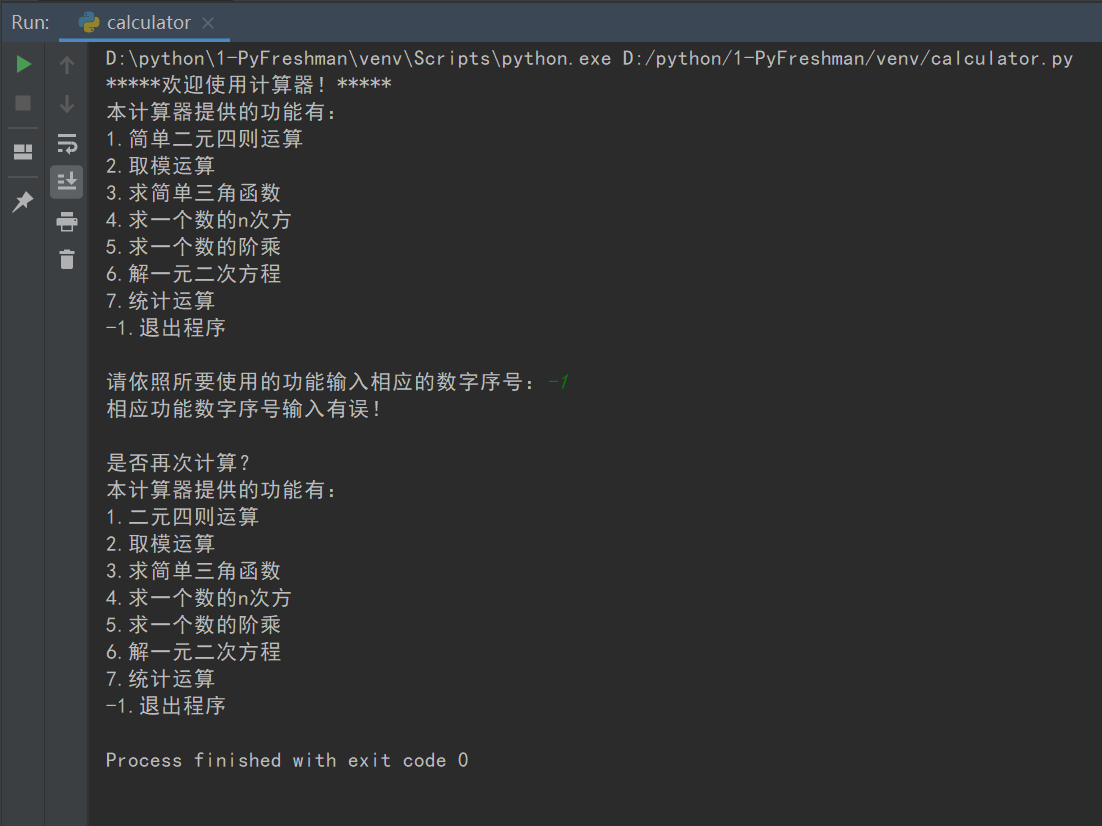• 问题3解决方案：在结构框架中加入

    elif t=='-1':
exit(0)


## 其他（感悟、思考等）

• 编码代码是学习一门特别有效的方法，应用使得所有死在纸面上的知识鲜活起来，使得学习更有获得感。
• 每一个程序的设计都需要不断的测试与改进。纵使只是一个小小的计算器程序也有许多东西需要考虑，需要涉及。
• 每次编程前都应该对目的、思路进行一个具体的描述与设计，这样可以保证编程的顺利与相对高效。

## 参考资料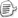posted on 2020-04-12 13:40  20191113林紫欣  阅读(327)  评论(0编辑  收藏